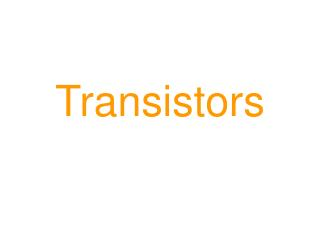DownloadDownload PresentationTransistors

# Transistors

Download Presentation## Transistors

- - - - - - - - - - - - - - - - - - - - - - - - - - - E N D - - - - - - - - - - - - - - - - - - - - - - - - - - -
##### Presentation Transcript

1. Transistors

2. Introduction • Transistors are : • Electronic switches • Amplifiers of electrical current • Many sensors (e.g. LDR, Thermistor, etc) only provide a very small output current or voltage. • To provide a practical working circuit, it may be necessary for a designer to amplify (e.g. make bigger) this current or voltage in order to drive an output device (e.g. Bulb, LED, Buzzer, etc).

3. There are two types • Bipolar Transistors (NPN or PNP) • Field Effect Transistors (FET) Note … We have only got to know about Bipolar NPN Transistors. Transistors are semiconductor devices. An NPN transistor is made from three layers of n-type and p-type semiconductor material.

4. Case Appearance Transistors come in a variety of cases:

5. Transistor Leads • A transistor has three leads which are called: • The Base (b) (responsible for activating the transistor) • The Collector (c) (The positive lead) • The Emitter (e) (The negative lead) • It is important that each of the leads is correctly identified C B E E B C

6. Transistor circuit symbol

7. How does a Transistor work? • For a transistor to work it has to receive a small voltage (e.g. 0.6 - 0.7 Volts) at its base. • This small voltage will result in a small electrical current to flow into the base and out of the emitter. • The resistance between the collector and emitter will fall. • A larger current will then flow from the collector to the emitter.

8. Amplification of current • The amplification of a transistor is known as the GAIN. • Two current paths flow through a transistor: • The base current which is small • The collector current which is large • The gain of a transistor is found by dividing the collector current by the base current and is represented by the symbol hFE.

9. Example 1 Calculate the gain of a transistor if the : collector current is 100mA when the base current is 0.5 mA Example 2 The hFE gain of a transistor is 40 when the collector current is 150 mA. Calculate the base and the emitter current Transistor Calculations

10. A Transistor Circuit E G A F D B C

11. Questions on the transistor circuit • Which of the components is the INPUT? • Which components are the output? • Which two components make up the potential divider? • What does component C do? • What is component D and how does it work? • What does component E do?

12. Single transistor circuit

13. Two transistor circuit • By adding a second transistor, the performance of the circuit is improved. • The second transistor benefits from the gain of the first transistor and the circuit becomes more sensitive to changes at the input. Darlington Pair • A common method of connecting two transistors is called a DARLINGTON PAIR or DARLINGTON DRIVER. The total gain is the gain of the first transistor multiplied by the gain of the second transistor.

14. Two transistor circuit(Darlington Pair)